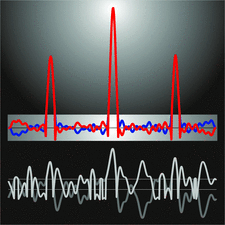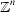# issue contentsFOUNDATIONSADVANCES
ISSN: 2053-2733

# November 2006 issueCover illustration: Determination of a one-dimensional structure by the charge flipping algorithm. Bottom curves: grey is the initial electron density obtained with a random choice of structure-factor phases; white is the result of charge flipping, i.e. the sign of the density below a threshold is reversed. Top curves: electron density after convergence (blue) and its flipped counterpart (red). Only the three atomic regions correspond to the true structure, small oscillations do not. See Oszlányi & Süto [Acta Cryst. (2004), A60, 134-141; (2005), A61, 147-152].

## research papers

### Orthorhombic sphere packings. I. Invariant and univariant lattice complexes

All homogeneous sphere packings were derived that refer to the invariant and univariant lattice complexes with orthorhombic symmetry. Examples of corresponding crystal structures are given.

### Discrete tomography of planar model sets

A special class of planar mathematical quasicrystals, i.e. the class of the so-called cyclotomic model sets, is introduced and the main algorithmic problems of the discrete tomography of these sets are discussed.

### Precession electron diffraction 1: multislice simulation

A multislice method for simulating precession electron diffraction data is presented, and simulated data are compared to experimental data for two materials systems. Dynamical diffraction effects are present in precession data but are somewhat smaller and intensity ordering is better preserved than in conventional transmission electron diffraction. Some of the implications of precession for conventional direct methods are discussed.

### Finite size effects of nanoparticles on the atomic pair distribution functions

The finite size effects of nanoparticles on the atomic pair distribution function (PDF) are calculated; these are essential to the structural analysis of nanoparticles.

### Indices of coincidence isometries of the hypercubic latticeExplicit formulas for the indices of certain coincidence isometries of the hypercubic lattice are obtained by analyzing the normal form of a rational orthogonal matrix.

### Resonant scattering and multiple Bragg X-ray diffraction in LaMnO3; a classical view

It has been possible to decide, by making use of multiple Bragg scattering, between two competing models for the d-electron density lobes in LaMnO3.

## book reviews

### International tables for crystallography, Vol. G: Definition and exchange of crystallographic data

Acta Cryst. (2006). A62, 464
doi: 10.1107/S010876730602616X